GMAT  >  Pie Charts

# Pie Charts Notes | Study Integrated Reasoning for GMAT - GMAT

## Document Description: Pie Charts for GMAT 2022 is part of Pie Chart for Integrated Reasoning for GMAT preparation. The notes and questions for Pie Charts have been prepared according to the GMAT exam syllabus. Information about Pie Charts covers topics like Learning Objectives, Introduction, Practice Question:, Significance of Pie Charts, Practice Question: and Pie Charts Example, for GMAT 2022 Exam. Find important definitions, questions, notes, meanings, examples, exercises and tests below for Pie Charts.

Introduction of Pie Charts in English is available as part of our Integrated Reasoning for GMAT for GMAT & Pie Charts in Hindi for Integrated Reasoning for GMAT course. Download more important topics related with Pie Chart, notes, lectures and mock test series for GMAT Exam by signing up for free. GMAT: Pie Charts Notes | Study Integrated Reasoning for GMAT - GMAT
 1 Crore+ students have signed up on EduRev. Have you?

Learning Objectives

- You will get an understanding of what are Pie Charts and a first-hand view of how data is presented through its organisation in the form of Pie charts.

- You will get an understanding of typical data which can be represented through Pie charts. Also, understand the concept of how the distribution of a whole is done in the form of Pie Charts.

- You will get a first hand feel of the typical question types that are asked on the basis of Pie charts.

- Practice exercise on Pie charts containing simulated examination questions

Introduction

Pie charts are specific types of data presentation where the data is represented in the form of a circle. In a pie chart, a circle is divided into various sections or segments such that each sector or segment represents a certain proportion or percentage of the total. In such a diagram, the total of all the given items is equated to 360 degrees and the degrees of angles, representing different items, are calculated proportionately. The entire diagram looks like a pie and its components resemble slices cut from a pie. The pie chart is used to show the break-up of one continuous variable into its component parts.

The types of pie charts:

There are two approaches to constructing a pie chart from any given data:

(A) Degree Approach:

The central angle in a circle represents 360°, so any part or segment in a pie chart is calculated as a proportion of 360°.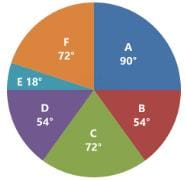(B) Percentage Approach:

In this case, any part or segment in a pie chart is calculated as a part of 100%.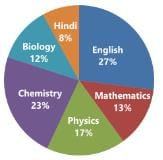Conversion of degree approach to percentage approach: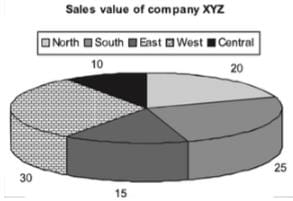If we convert the same pie chart into the degree format, we will be required to do the following conversions:
Total = 100%
Hence, 1% = 3.6°
Central = 10% = 36°
North = 20% = 72°
South = 25% = 90°
East = 15% = 54°
West =30% = 108°

Practice Question:

Question for Pie Charts
Try yourself:The central angle of a sector is 72º. What percentage of the circle is comprised by the sector?

Significance of Pie Charts

The pie chart has gained prominence due to the following reasons:

- In a pie chart, we get a clear picture of the contribution of different sectors to the build up of the total. E.g., presentation of budgets.

- Comparing two pie charts is easier than comparing two bar charts or any other format of data representation.

Example:

Following is the cost analysis of a book “Guide to Digital Marketing”.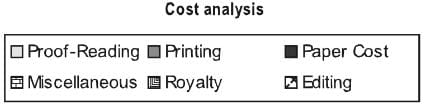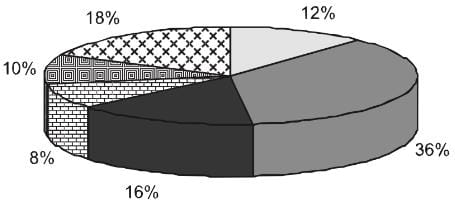What is the central angle showing the cost of paper?
(a) 42.8°
(b) 32.6°
(c) 36.8°
(d) 57.6°
Sol: Percentage of paper cost in total cost - 16%
We know that the sum of angles in pie chart is 100°
Hence, Required Angle = (16/100 × 360) = 57.6°

Practice Question:

Question for Pie Charts
Try yourself:If 5500 copies of the book are published and the miscellaneous expenditure amounts to Rs.36,960 and the marked price is 40% above the cost price, then the marked price of each copy is

The document Pie Charts Notes | Study Integrated Reasoning for GMAT - GMAT is a part of the GMAT Course Integrated Reasoning for GMAT.
All you need of GMAT at this link: GMAT

## Integrated Reasoning for GMAT

19 videos|28 docs|24 tests
 Use Code STAYHOME200 and get INR 200 additional OFF

## Integrated Reasoning for GMAT

19 videos|28 docs|24 tests

Track your progress, build streaks, highlight & save important lessons and more!

,

,

,

,

,

,

,

,

,

,

,

,

,

,

,

,

,

,

,

,

,

;